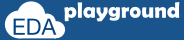## Contact / Report an issue

### Jump Statements:

#### break

Execution of break statement leads to end of the loop.

#### Example-1: break

```#include "systemc.h"
int sc_main (int argc, char* argv[]) {

//break-example
for(int i=0;i<8;i++) {
cout <<" Value of i="<<i<<endl;
if(i == 4) {
cout <<" Calling break"<<endl;
break;
}
}

// Terminate simulation
return 0;
}
```

#### Simulator Output:

j=0 i=4
j=1 i=3
j=2 i=2
j=3 i=1
Execute the above code on#### continue

Execution of continue statement leads to skip the execution of statements followed by continue and jump to next loop or iteration value.

#### Example-1: continue

```#include "systemc.h"
int sc_main (int argc, char* argv[]) {

//continue-example
for(int i=0;i<5;i++) {
cout <<"Before continue, value of i="<<i<<endl;
if((i > 1) && (i < 4)) {
cout <<"     Calling continue"<<endl;
continue;
}
cout <<"After  continue, value of i="<<i<<endl;
}

// Terminate simulation
return 0;
}
```

#### Simulator Output:

Before continue, value of i=0
After continue, value of i=0
Before continue, value of i=1
After continue, value of i=1
Before continue, value of i=2
Calling continue
Before continue, value of i=3
Calling continue
Before continue, value of i=4
After continue, value of i=4
Execute the above code on#### Example-1: goto

```#include "systemc.h"
#include <iostream>
using namespace std;

int sc_main (int argc, char* argv[]) {
//declaration and initialization
int a = 15;
int b = 10;

my_lable:
cout <<" @ my_label "<<endl;

if( a > b ) {
cout <<" a is greater than b a = "<<a<<" b = "<<b<<endl;
a--;
goto my_lable;
}
else
cout <<" b is greater than a "<<endl;

// Terminate simulation
return 0;
}
```

#### Simulator Output:

@ my_label
a is greater than b a = 15 b = 10
@ my_label
a is greater than b a = 14 b = 10
@ my_label
a is greater than b a = 13 b = 10
@ my_label
a is greater than b a = 12 b = 10
@ my_label
a is greater than b a = 11 b = 10
@ my_label
b is greater than a
Execute the above code on